All submissions of the EM system will be redirected to Online Manuscript Submission System. Authors are requested to submit articles directly to Online Manuscript Submission System of respective journal.

# Original Article

, Volume: 6( 2)

## Theory of the Relative Image

*Correspondence:
Moawad EY , Department of Engineering, Ain Shams University, 217 Alhegaz Street, Alnozha, 11351, Cairo, Egypt, Tel: +20226831474; E-mail: emadmoawad@hotmail.com

Received: June 06, 2017; Accepted: August 20, 2017; Published: August 25, 2017

Citation: Moawad EY. Theory of the Relative Image. J Space Explor. 2017;6(2):126

### Abstract

From the beginning, the entire contents of the universe have been moving along paths seemed elliptically under the action of the gravitational forces that appeared unlike the reality; constant and always attractive. Such perception about the gravitational forces misled the latter to imply that our universe is either expanding or contracting associated with orbital decay narrowing these elliptical paths gradually until collapse. Conversely, theory of the relative image proves that the gravitational forces are variable elastic restoring forces under which the cosmological objects move simple harmonic motion along wavy paths. The total work done along these wavy paths is zero without energy loss preserving the fixed distances between those bodies in renewable frictionless system. This network of spring forces acting on the cosmological contents was installed by distributing the matter evenly along the spacetime of the universe. Equilibrium of the cosmological body is inseparable from the equilibrium of our static universe in which velocities of all its contents are not constant but accelerated and then decelerated in an isolated system of permanent self-sustaining.

### Keywords

Gravitational forces; Doppler effect; Black holes; Cosmic microwave background radiation; Gravitational waves; Big bang

### Introduction

Today scientists describe the universe in terms of two basic partial theories – the general theory of relativity and quantum mechanics which are known to be inconsistent with each other as they cannot both be correct . Einstein’s theory has followed the classical view starting from Kepler regarding the motion of the moon around the earth and the planets around the sun following elliptical paths. In recent years the even smaller deviations of the orbits of the other planets from the Newtonian predictions have been measured by radar and found to agree with the predictions of general relativity . Afterwards, theory of Quantum mechanics has come out dealing with phenomena on extremely small scales, such as a millionth of a millionth of an inch and no longer describes the real world in terms of particles and waves . For some purposes, it is helpful to think of particles as waves and for other purposes it is better to think of waves as particles. Quantum mechanics tells us that all particles are in fact waves and that the higher the energy of a particle, the smaller the wavelength of the corresponding wave . The electromagnetic attraction between negatively charged electrons and positively charged protons in the nucleus causes the electrons to orbit the nucleus of the atom as the gravitational attraction causes the earth to orbit the sun. The only areas of physical science into which quantum mechanics has not yet been properly incorporated are gravity and the large-scale structure of the universe. On the other hand, the discovery that the universe is expanding was one of the great intellectual revolutions of the twentieth century . It explains the nonexistence of absolute rest so that one could not give an event an absolute position in space or determine whether two events that took place at different times occurred in the same position in space. For example, an observer could use radar to say where and when an event (like a planet position) took place by sending out a pulse of light or radio waves. Part of the pulse is reflected back at the event and the observer measures the time at which he receives the echo. During that time, the observer on the earth was not at rest due to motion of the earth in its path. Thus, the it could neither be said that time of the planet position was at halfway between when the pulse was sent and the time when the reflection was received back nor its distance from earth is half the time taken for this round trip, multiplied by the speed of light. Similarly, the elliptical orbits of the planets predicted by general relativity which were almost exactly the same as those predicted by the Newtonian theory of gravity were traced by ignoring the fact of the nonexistence of absolute rest for the observers and the planets’ positions. The effect of this fact is clear in carrying out experiments with moving bodies like playing Ping-Pong on the moving train; one would find that the ball falls vertically downwards like a ball on a table by the track. The Ping-Pong ball on the train bounces straight up and down, hitting the table twice on the same spot that moves with the train. To someone on the track, motion of the spot would be observed as the two bounces would seem to take place in different spots distant apart by the distance traveled by the spot with the train during the time between the two bounces. Accordingly, the path of the Ping-Pong ball that seems vertical straight line to the observer on the train also seems a curved path of a projectile of velocity equivalent in magnitude and direction to that of the train falling down after reaching the maximum height to another observer at rest with respect to the train on the track. Figure. 1 shows path of the Ping-Pong ball as shown for two observers one on the train and other on the track.

Figure 1: Path of the Ping-Pong ball as shown for two observers one on the train and other on the track.

This clarifies the effect of the relative velocity between the vertical plane of the observer’s ray of sight and the path of the moving body on the determined image of the motion. On the train, there is no relative velocity between the vertical plane of the observer’s ray of sight and the path of the ball, whereas on the track the relative velocity between them is the velocity of the train.

Despite both images are for one motion different in all physical quantities, like position vectors, displacement, velocity, direction of motion, or, both images are correct relative to their observers. Thus, we should consider the fact of the relative image as the companion of the other fact of the nonexistence of absolute rest by taking into account the relative velocity between the source of the image (path of the ball) and the observer. Similarly, the path of the moon with respect to earth or that of any other planet of our solar system with respect to the sun seems elliptical path relative to either of the observer on earth or that on the other planet. Thus, once the relative velocity between the observed object and the observer changes, the traced image would be changed as well to another relative image different from the elliptical one has its own physical quantities. Relative images for the same motion with speed less than that of light differ in length and velocity of the motion but have the same time of incidence or observation according to the concept of the absolute time of the classical physics. While relative images of the same motion with speed of light differ in length and time of incidence but have the same speed of light according to the theory of relativity. As the relative velocity between the moon and earth (1023 km/s ) differs from that between the moon and the sun (2.97×104 km/s ), then suppose a brave astronomer on a spaceship orbiting nears the sun, using x-ray detector would trace an image for the path of the moon with respect to the earth different from the elliptical image that traced by the observer on the earth along the same periodic time. The moon would seem to that astronomerorbiting the sun-moving with the relative velocity between the sun and the moon which is the velocity of the earth (2.97×104 km/s ) like our Ping-Pong ball that seems to the observer on the track moving with the relative velocity between the track and the Pin-Pong ball which is the velocity of the train. Unlike the Ping-Pong ball that seems follow a path of a projectile to the observer on the track, instead the moon seems follow a wavy path of an oscillator to the astronomer on his/her spaceship orbiting the sun, since the moon doesn't fall down to hit the earth but preserves the fixed distance from the earth along its motion. Notably, the wave path of the relative image determined by the astronomer on his/her spaceship orbiting nears the sun using x-ray detector as will be explained in the discussion of periodic time and amplitude equivalent to the periodic time and the radius of the elliptical image. Figure. 2 shows the wavy image of the path of the moon (silver) with respect to the earth (blue) as determined by an astronomer her/his spaceship orbiting nears the sun.

Figure 2: The wavy image of the path of the Moon with respect to the Earth as traced from the Sun.

Figure. 3 shows the elliptical image of path of the moon with respect to the earth as determined by the observer on the earth.

Figure 3: The apparent elliptical image of the path of the Moon with respect to the Earth as observed from the Earth.

Experimental

The path of the earth or any other planet of our solar system with respect to the sun that traced elliptically from the earth or the other planet is traced wavy from the sun. Consequently, the non-existence of the absolute rest in our universe is responsible for the relative images for every event so that no real image is available in our universe. Accordingly, concept of the relative image should be taken into consideration in interpreting everything concerning our universe.

Theory of the Relative Image

Image of the straight path of a moving body in the absence of relative velocity between the observer and the body is a curved path in the presence of relative velocity between the body and the observer

Going back to the experiment shown in Figure. 1 there is relative velocity between the observer on the track and the Ping-Pong ball equivalent to the velocity of the train (u=40 m/s). The Ping-Pong ball fells down and hits the spot in the train after 1 second. Figure. 4 shows the parabolic trajectory of the ball seemed to the observer on the track similar to that of a projectile after reaching the maximum height and falling down under the effect of gravity. The Cartesian equation of the path of the Ping-Pong ball (projectile p) shown in Figure. 4 is given by:

Figure 4: The path of a projectile p of initial horizontal velocity u under the effect of gravity in interval T sec.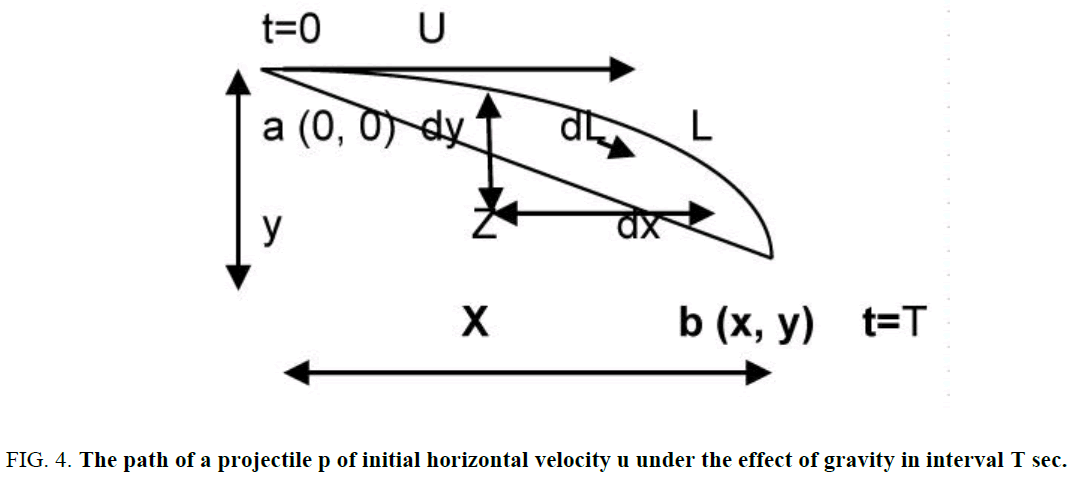andwhere x=ut, dx=u dt and y=0.5 gt2 and g is the gravity acceleration. Accordingly, the displacement vector () of the final position of the spot (ut m, 0.5gt2 m) is (40 m, 4.9 m) of magnitude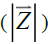40.299 m using the Pythagorean Theorem in Euclidean space.

While, the arc length (L) of the path from point (a) to point (b) is given by:(1)

While from equation 1 the arc length of the path from the initial to final positions is given by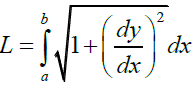. Then by substitution forx=ut, dx=u dt, at x=a m t=0 sec and at x=b m t=T sec in equation 1 the arc length of the path along the period of T sec is given by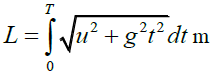As our Ping-Pong ball is seemed to the observer on the track to be projected horizontally by due to the relative velocity of the train (u=40 m/s) to hit the spot in 1 sec thenaccording to theory of the relative image. The increase of L (the path between two points on curve) than(the short distance of line segment connecting the two points on the curve) confirms that our Ping- Pong ball followed the proposed parabolic trajectory of a projectile in the observed image from the track.

Image of the elliptical path of orbiting body around another body in the absence of relative velocity between the observer and the other body is a wave path of simple harmonic motion in the presence of relative velocity between the observer and the other body

Figure. 1 shows two paths along which the moon moves as detected by the observer that orbits nears of the sun. The first is a wavy path of simple harmonic motion around the path of the elliptical path of the earth around the sun. While the second is the elliptical path of the earth around the sun along which the moon orbits the sun as a companion for the earth. According to the classical physics, the mathematical representation of the position of the moon with respect to these paths shown in Figure. 2, as a function of time (t) is (Y= Asinωt and X = ut ), where A is the amplitude,is the angular frequency, T is the periodic time and u is the relative velocity of the moon with respect to the observer at the sun which is the same as that of the earth (2.97×104m/s) . While from equation 1 the arc length between any two points a, b on this path is given by:(2)

Regarding the motion of the moon shown in Figure. 2 and Figure. 3 as observed in with different relative velocity between the observer and the moon respectively similar to that of the Ping-Pong ball shown in FIG.1 as observed from the track and from the train. Thus, as the Ping-Pong ball is seemed to the observer on the track moving with the velocity of the train, the moon is seemed to the astronomer on the spaceship orbiting nears the sun moving with the velocity of the earth (i.e. u=2.97×104 m/s ). From Figure. 2 the amplitude (A) of the wave motion of the moon with respect to the earth is 3.844×108 m, whereas T is 227.321661 d . Accordingly, from equation 2, the wavelength of the moon as observed by the astronomer on the spaceship orbiting nears the sun is ( λ = ut ) 7.015×108 m. On the other hand, the mathematical representation of the oscillator wave function (Ψ ) that determines the position of the oscillator (moon) with respect to the path of the earth around the sun according to the quantum physics for the simple harmonic motion at the ground state is given by that determines the position of the oscillator (moon) with respect to the path of the earth around the sun according to the quantum physics for the simple harmonic motion at the ground state is given by, where ? =A (Amplitude) at the normalization condition (0, A) . Thus, length of the wave function (L) is given by:(3)

By knowing the periodic time (T) and the amplitude (A) shown in Figure. 7 of the simple harmonic motion of the moon which are 27.321661 d and 3.844×108 m respectively, m is the mass of the moon (7.324 ×1022 kg),, λ is the wave length shown in Figure. 7 and (h) is Plank’s constant, then (L) is 7.031 × 1010 m (equivalent to λ ) identical and confirming to that derived previously through the classical mechanics.

Figure. 5 represents the wave function of the oscillator (moon) with respect to the earth as observed by the astronomer on the spaceship orbiting nears the sun.

Figure 5: The quantum oscillator wave function of the Moon motion with respect to the Earth as observed from the Sun.The quantum oscillator wave function of the Moon motion with respect to the Earth as observed from the Sun.

The other observer on earth determines that the moon travels a distance of (1023 m/s × 27.321661× 86400 s) 2.41488512 × 109 m on elliptical path during the same periodic time according to the gravitational Newtonian theory. Accordingly, the wavelength of the wavy image (λ ) is longer than the length of the elliptical path with radius r ( 2π r ) by the ratio of the relative velocities between the moon and the two observers on the sun and the earth respectively (i.e..

Since the two images are for a motion of one body during the same period then the energy of the body should be the same in both images. The moon of mass (m=7.324 × 1022 kg) and orbiting with velocity (v=1023 km/s) in the image of the elliptical path around the earth (Figure. 7) of radius (r=3.844 ×108 m), with kinetic energyof 3.83×1028 j . On the other hand, the total energy (E) of the moon that oscillates in the image of the wavy path around the elliptical path of the earth around the sun (Figure. 7) with displacement (x; of maximum value of A) from the equilibrium position which is the elliptical path of the earth around the sun forms a wave of amplitude (A=3.844 ×108 m) with angular velocity ω=2π/t=2.66169953 10-6Hz) is ½KA2 where k=ω2 m is the constant of the restoring elastic force exerted on the oscillator) 3.837×1028 j. Thus, the energy of the moon along the image of the wavy path around the elliptical path of the earth around the sun observed by the astronomer on the spaceship orbiting nears the sun is 100% identical to that in the image of the elliptical path around the earth observed by the observer on the earth. On the other hand, the exerted constant force acting on the moon in the image of the elliptical path around the earth is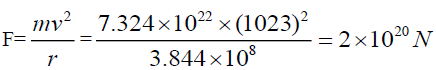, whereas the variable force acting on the moon in the image of the wavy path around the elliptical path of the earth around the sun (F=- kx; with maximum value at x=A) is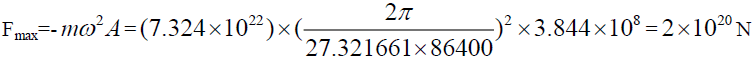which is also 100% identical to that of the moon in the image of the elliptical path around the earth. Similarly, an observer from the earth or nears the sun observes the earth moves along an elliptical path around the sun of radius 1.496×1011 m with velocity 2.97×104 m/s with periodic time of 3.156×107 s and orbital length 9.39965×1011 m. . While another brave astronomer on her/his spaceship orbiting nears the Milky-Way observes the earth moves along two paths; the first is a wavy path around the elliptical path of the sun around the Milky-Way of amplitude (A) 1.496×1011 m with velocity 2.97×104 m/s with periodic time of 3.156×107 s and the second is the elliptical path of the sun around the Milky-Way along which the earth orbits the Milky-Way as a companion for the sun so that the earth is seemed to the astronomer moving with the velocity of the sun (i.e. u= 2.2×105 m/s) with periodic time of the galactic period ( 2.44×108 × 3.156×107 s) . Accordingly from equation 2, the wavelength of the earth around the elliptical path of the sun around the Milky-Way observed by the brave astronomer at the Milky-Way is ( λ = ut ) 12 6.9432×10 m. Accordingly, the wavelength of the wavy image (λ) is longer than the length of the elliptical path with radius r ( 2π r ) by the ratio of the relative velocities between the earth and the two observers on the sun and the earth respectively according to equation 4 (i.e.(4)

Image of the wavy path of oscillator around the path of second body in the presence of relative velocity between the first observer and the second body is a wave path of longer wavelength in the presence of relative velocity between the first and the second observers

From the above, image of the motion of the moon around the earth is detected elliptically and wavy by two observers from the earth and the sun respectively. There is no relative velocity between the earth and the first observer, whereas between the earth and the second observer there is relative velocity of 2.97×104 m/s which is the velocity of the earth with respect to the sun. Then the velocity of the earth with respect to the sun makes the observer at the sun to see the path of the moon in the form of a wave instead of elliptical as seen from the earth. Let us now study the effect of the velocity of the wave source (that causes the wave image) on the properties of the formed wave image. Suppose a third observer detects using radar the same motion of the moon with respect to the sun from a third position of relative velocity with respect to the sun like the center of our galaxy which is the Milky-Way. According to equation 2, the relative velocity (u) of the sun with respect to the Milky- Way ( 2.2×105 m/s) makes the observer at the Milky Way to see the wave path of the moon of length (L)=dt=2.2×105 × 27.321661×86400(i.e. u ×T=λ )=5.19335726 ×1011 m. This value of the wavelength of the wave motion of the moon with respect to the sun as determined from the Milky-Way differs from that with respect to the earth as determined from the sun. The effect that causes that difference in the determined wavelength in the two observations of the same motion is the difference in the relative velocity of the wave source with respect to the observer in each case. According to the classical physics the effect of the relative velocity of the observer with respect to the wave source on the observed image is consistent with a well-known phenomenon called Doppler Effect ; for the observer at the sun, the earth is the source of the moon wave, whereas the sun is the source of the moon wave for the observer at the Milky-Way. Thus, by comparing the determined wavelengths of the same wave motion of the moon by the two observers from the sun and the Milky-Way to the relative velocities of the earth and the sun (sources of the wave motion of the moon) with respect to theobserver in either case, we find that(5)i.e. the determined wavelength is proportional to the velocity of the wave source, whereas the periodic time (T) is constant in the two images as(6).

The property expressed in equation 5 is for all kinds of waves including light waves as the wavelength is proportional to the velocity of the wave source but not to the wave velocity that may be the speed of light. This is similar to the effect you get when a train passes, and its whistle goes from high to low pitch as it goes past you. When this effect is applied to light waves as opposed to sound waves, it makes light that is emitted by something moving away from us (seems faster) appear more red of longer wavelength, and light emitted by something moving towards us (seems slower) appear more blue of shorter wavelength. In consistent with the theory of relativity, the difference in the effect of the velocity of the wave source on the light wave (or any type of the electromagnetic waves that propagate with speed of light) from that on other kinds of waves is the decrease in the periodic time of the light wave when the wave length increases due to the increase of the relative velocity of the wave source and vice versa following: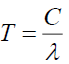(7), where C is the speed of light. Thus, theory of the relative image is consistent with the classical and modern physics.

The mechanism of the gravitational force and the balance of the universe

Theory of the relative image provides a perception about the mechanism by which the gravitational forces act on the cosmological bodies achieving the equilibrium of our universe. Each body moves around a second one along a path has different detected shape according to the relative velocity between the observer and the second body. In the absence of this relative velocity or when the observer detects the first body orbiting the second body from the second body itself (1st image), this path appears elliptical path and the second body appears stationary lies on its center. The force acting on the first body in the 1st image appears constant centripetal force and always attract the first body towards the second one at the center of the elliptical path and equivalent to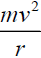, where (m), (v) are the mass and velocity of the first body and (r) is the radius of the elliptical path as already known by the latter and confirmed by the former. In the presence of relative velocity between the observer and the second body (2nd image), the path of the first body appears wavy while the second body appears moving along another elliptical path around a third body. The elliptical path appears to the second observer in the 2nd image is the axis of the wavy path of the first body. The amplitude (A) and periodic time (T) of the wavy path in the 2nd image are equals to the radius (r) and (T) of the elliptical path detected by the first observer in the 1st image. Accordingly, the first body appears in the 2nd image has two motions; the first along a wavy path alone and the second as a companion for the second body along an elliptical path orbiting the third one. Figure. 6a and Figure. 6b show the 1st and 2nd images respectively for the first body (silver) orbits a second one (blue) in the absence and presence of relative velocity between the observer and the second body respectively.

Figure 6b: (2nd image) (Observer on the second body (blue)) (Observer on the third body (yellow) around which the second body (blue) orbits)

These two motions of the first body in the 2nd image is under the action of two forces; the first is variable elastic restoring spring force under which the first body moves in simple harmonic motion along the wavy path towards and away from the elliptical path around which the second body orbits the third one. The magnitude and direction of this spring force is given by F=-kx, where (k) is the elastic restoring constant and (x) is the distance between the first body and the elliptical path around which the second body orbits the third one. The negative sign indicates that (F) is in the opposite direction to that of the increase of (x). The maximum value of this force (Fmax=-mω2A) is at x=A. The second force under which the first body appears moving as a companion for the second body along the elliptical path in the 2nd image is a constant centripetal force appears always attract both bodies as a one unit towards the third body at the center of the elliptical path similar to that appeared acting on the first body in the 1st image. Similarly, the appeared motion for the first and second bodies in the 2nd image along the elliptical path orbiting the third body is detected from the third body in the absence of relative velocity between the second observer and the third body. Accordingly, in the presence of relative velocity between a third observer and the third body the elliptical path that seemed to the second observer in the 2nd image is a wavy path in a 3rd image along which the second body moves under variable spring forces. While the wavy path appeared in the 2nd image along which the first body moves is a wavy path of longer wave length with the same spring forces in the 2nd image. Accordingly, in the orbiting systems the gravitational force acting on the body orbits a second one is a variable force of reversible direction similar to the spring forces of the simple harmonic motion. Under which the orbiting body moves along a wavy path observed only in the presence of relative velocity between the observer and the second body. Such mechanism of the gravitational forces acting on the cosmological bodies is the perception of theory of the relative image through which the equilibrium of our universe has been achieved. For instance, in the Earth-Sun system; the moon orbits around the earth under the gravitational force acting on the moon by the earth. The path of this motion is detected elliptically from the earth under a constant centripetal force. The same path is detected from a place of relative velocity with the earth like the sun wavy, under a variable spring force (Figure. 7). Figure. 7 shows the motion of the moon (silver) along a wavy path (silver) alone and along elliptical path (blue) as a companion for the earth (blue) around the sun (yellow) as observed from the sun.

Figure 7: The motion of the Moon (silver) along a wavy path (silver) alone and along elliptical path (blue) as a companion for the Earth (blue) around the Sun (yellow) as observed from the Sun.

The restoring elastic (spring) force acting on the moon along its wavy path around the earth is given by-Kx=-k A sin ωt of maximum value of-KA, where A is the amplitude of the simple harmonic motion equals to the fixed distance between the earth and the moon (3.844×108 m). This force varies according to the distance (x) between the moon and the elliptical path of the earth around the sun. The negative sign indicates that the direction of the spring force is opposite to the increase direction of the displacement (x) of the moon from the equilibrium position which is the elliptical path of the earth around the sun. From Figure. 7 on the positions of intersection of the wavy and the elliptical paths, the moon acquire its extreme velocity (ωA=1023 m/s) and is driven by maximum deceleration (-ω2 A) whereas the spring force acting on the moon vanishes as the moon is on the equilibrium position of the spring force (x=0) which is the path of earth around the sun. At those points of intersection between the two paths (x=0) (when the spring forces vanish), the centripetal forces acting on the moon (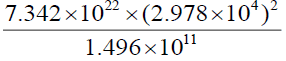) and the earthby the sun are 4×1020 N, 3.54×1022 N respectively of summation of 3.58×1022N. While at positions of troughs and crests, (x=A,-A) shown in Figure. 7 (when t=T/4 and 3T/4) the moon velocity instantly vanished, the restoring elastic (spring) force acting on the moon becomes maximum (KA=2×1020 N ) that accelerates the moon once again in direction of the wavy path. Thus, along the whole wavy path the variable spring force acting on the moon is(-mω2Asin ωt) and ∈[0→2×1020N]) causes the moon to oscillate in the wavy path below and above the elliptical path of the earth around the sun. When the spring force acting on the moon is opposite to the direction of the centripetal forces acting on the moon and the earth by the sun, the moon goes down the elliptical path along the wavy path until the trough (x=A) at which the resultant of forces acting on the moon and the earth decreases to 3.56×1022 N only. Afterwards, the spring force acting on the moon decreases again (x< A), while the resultant force acting on the moon increases gradually to 3.58×1022 N at (x=0) once again. When the spring force acting on the moon is in the direction of the centripetal forces acting on the moon and the earth by the sun, the moon goes up the elliptical path along the wavy path until the crest (x=-A) at which the resultant of forces acting on the moon and the earth increases to 3.60×1022 N. Afterwards, the spring force acting on the moon decreases again (x>-A), while the resultant force acting on the moon decreases gradually to 3.58×1022 N at (x=0) and so on. Accordingly, the resultant force acting on the moon and the earth by the sun along the whole elliptical path around the sun is not of constant value as postulated by the Newtonian gravitational theory but varies as proved by theory of the relative image with average ( 3.58×1022 N) equivalent to that predicted by the Newtonian gravitational theory. Similarly, the earth orbits around the sun under the gravitational force acting on the earth by the sun. The path of this motion is detected elliptically from the sun under a constant centripetal force. The same path is detected from a place of relative velocity with the sun like the Milky-Way wavy under a variable spring force. Figure. 8 shows the moon (silver) and the earth (blue) move along the elliptical path (brown) around the Milky- Way (not shown) as companions for the sun (yellow), and along wavy paths (silver and blue respectively) around the sun as observed from the Milky Way.

Figure 8: The Moon (silver) and the Earth (blue) move along the elliptical path (brown) around the Milky-Way (not shown) as companions for the Sun (yellow), along wavy paths (silver and blue respectively) around the Sun as observed from the Milky Way.

In Figure. 8 each of the moon and the sun moves under the action of variable spring forces causes the moon and the earth to oscillate in wavy paths around the elliptical path of the sun around the Milky-Way. Each of these spring forces can be determined as previously shown for the motion of the moon around the earth. Thus, theory of the relative image negates that the gravitational forces acting on the cosmological bodies are constant and centripetal always attractive. Conversely, it proves that the gravitational forces that act on the cosmological bodies are variable elastic restoring spring forces under which the cosmological bodies move along wavy paths preserving the fixed distances between them which are the amplitudes of their simple harmonic motions.

Applications on the Theory of Relative Image Moon light is invisible and the moon is so dim couldn’t be seen by an astronomer orbits by her/his spaceship nears of the sun

Moonlight is the light that reaches Earth from the Moon, consisting mostly of sunlight, with some starlight and earthlight reflected from those portions of its surface which the Sun's light strikes. Then it comes to mind to ask, is this light can be seen by an astronomer at spaceship orbits nears the sun who determined (in the 2nd image) the wavelength of the wavy motion of the moon with respect to the sun? Determining the path of the moon doesn’t mean that the moon is visible as positions of the moon along its path are determined using a radar receives the reflected rays from the moon. The effect on the wavelength of the moon light is similar to that determined on the image of the wavy path of the moon in the 2nd image. The relative velocity of the wave source (the moon in the 1st image) with respect to the first observer (at the earth) is 1023 km/s, whereas the relative velocity of the wave source (the earth in the 2nd image) with respect to the second observer (the sun) is 2.97×104 m. Thus, from equation 5, the wavelength of the moon light when determined from the sun is longer29 times than that determined in the range of the visible light from earth in the 1st image. Accordingly, the moon light that appears to the earth in the 1st image is invisible and lies in the infrared range in the 2nd image with respect to the spaceship orbit near the sun. Thus, the moon is so dim and couldn’t be seen from the spaceship orbit near the sun and all that would be considered would be a black hole in space. Similarly, from earth; no visible light can be seen getting out of bodies moving by high relative velocity with respect to the earth and those bodies seem black holes in the space. While those black bodies with respect to the earth are visible and bright from other positions of lower relative velocities with respect to them. For instance, the moon light is invisible with respect to the observer near the sun as the relative velocity between the moon and the sun is 2.97×104 m/s, whereas visible from the earth as the relative velocity between the moon and the earth is 1023 km/s only. This provides a clear-cut criterion to accept the hypothesis of the effect of the relative velocity of the wave source with respect to the observer on the wavelength of the detected wave.

Astronaut on the moon can see planets of the solar system but not closer stars

Venus is the second planet in the solar system closer to the Sun and the second brightest natural object in the night sky after the Moon . It travels at an average speed of 126,077 kilometers per hour (35 km/s) in its orbit anticlockwise (like earth) around the Sun . From Figure. 9 as detected by an observer orbits nears the sun; the moon moves in a wave path perpendicular to the path of the earth around the sun that parallel to that of Venus. Thus, the relative velocity is between Venus and the earth is the same as that between Venus and the moon. Venus (wave source) appears to the observer on earth by the naked eye as a white point of light brighter than any other planet or star (apart from the Sun) . Then the astronomer at the moon is expected to see the same view of Venus (wave source) by the naked eye. FIG. 9 shows the relative image of Venus and Sirius with respect to the earth and the moon as observed from the sun. While, Sirius is a star system 10 times larger than our sun, twice as massive and twenty times as much luminescence and the brightest star in the Earth's night sky . The spectrum of this star is a noticeable red shift despite of moving towards the sun (and consequently the earth) gradually at 5.5 km/s . The redshift of the spectrum occurs whenever a light source moves away from an observer or shifted to the red end of the spectrum of the visible light. Accordingly, Sirius’s light has long wavelength equivalent to a lower frequency and lower photon energy, in accordance with, respectively, the wave and quantum theories of light. In addition, Sirius emits a higher than expected level of infrared radiation, as measured by IRAS space-based observatory . While, The Chandra X-ray Observatory image shows Sirius B outshining its bright partner as it is a brighter X-ray source that characterized by much shorter wave length than that of the visible light . On the other hand, Sirius (the wave source) is moving with relative velocities 5.5 km/s with respect to the path of the earth (observer), and 1023 km/s with respect to the moon (observer) that moves along wavy path perpendicular to the path of the earth around the sun as shown in FIG. 9. Thus, from equation 5, the determined wavelength of Sirius emissions by on the moon is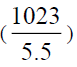186 times more than that determined on the earth due to the increase in the relative velocity of the wave source with respect to the observer. Accordingly, the light of the brightest red star in the Earth's night sky (Sirius) can be neither seen, nor other emissions (x-rays, infrared) can be detected from the moon.

Figure 9: The relative image of Venus and Sirius with respect to the Earth and the Moon as observed from the Sun.

Intensity of radiation emitted by black bodies differs by the change of the observer relative velocity

Objects at any temperature emit electromagnetic waves in the form of thermal radiation from their surfaces . The peak of the wavelength distribution shifts to shorter wavelengths as the temperature increases according to Wien’s law: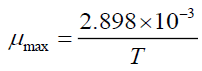where T is the absolute temperature of the surface of the object emitting the radiation . From equation 5, the wavelength of the detected electromagnetic radiation is directly proportional to the velocity of the wave source. Then color (wavelength) of the emitted light (electromagnetic radiation) is an observational evidence for each of the temperature of the surface of the wave source and the relative velocity between the observer and the wave source.

Accordingly, the detected radiation by the same object is also relative according to the relative velocity of the observer. For instance, the effective temperature of the Sun is 5778 K . Using Wien's law, an observer on earth of 2.97×104 m/s relative velocity with respect to the sun finds a peak emission per nanometer (of wavelength) at a wavelength of about 500 nm in the green portion of the spectrum near the peak sensitivity of the human. On the other hand, another observer orbits in the nears of the Milky-Way of 2.2×105 m/s relative velocity with respect to the sun finds using a radio telescope that the peak emission of the sun is at a wavelength of about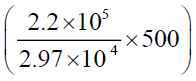3.703 μ m out of the visible light in the infrared portion of the electromagnetic spectrum. i.e. the astronomer orbits in the nears of the Milky-Way would detect by his/her radio telescope our sun as a black hole emits infrared rays. Furthermore, the change in the detected wavelength of a wave source (star) emission along time is an evidence for whether the star moves towards us when the emission blue shifted or receding from us when the emission redshifted. In the same time, the wavelength of light where a star's emission is concentrated is related to the star's temperature-the hotter the star, the more blue it is; the cooler the star, the more red it is. Thus, the relationship between the velocity of the wave source (star) with respect to the observer and the temperature of the emitted light is confirmed as well. Neutron stars which are usually observed from earth by Hubble telescope very hot and typically have a surface temperature around one million Kelvin at which most of the light generated by a neutron star is in Xrays . Such observations are confirmed using Wiens law as the wave length of the emitted radiation by such neutron star of surface temperature around one million Kelvin is 2.898 nm in the x-rays region. In the same time, such neutron stars are known to travel at speeds of hundreds of kilometers per second, with one shown in 2005 to be moving at 1100 km/s (see Fastest pulsar set to escape the Milky Way) . Thus, according to equation 5, an observer orbits near that neutron star would detect the maximum wavelength of sun’s emissions 18.53 μ m lies in the microwave region, while another observer orbits near the sun would detect the maximum wave length of the emissions of such neutron star 21. 409 nm lies in the ultraviolet region. However, using Wein’s law the detected temperature of the surface of such neutron star by the observer orbits near the sun would be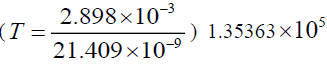Kelvin only. Thus, it can be said that the detected temperature of emitted radiations by bodies is also relative according to the relative velocity of the temperature source with respect to the observer. This result clarifies how colors and temperatures of the emitted light waves are always going to be the same along the periodic time of stars during which they appear move with constant velocity under the apparent effect of the gravitational forces in their image along their elliptical paths.

With a traditional optical telescope, the space between stars and galaxies (the background) is completely dark. While, a sufficiently sensitive radio telescope shows a faint background glow, almost isotropic, that is not associated with any star, galaxy, or other object. This glow is strongest in the microwave region of the radio spectrum and is well known by the cosmic microwave background radiation (CMBR), the fundamental observational cosmology because it is the oldest light in the universe . The Big Bang cosmology has explained the CMBR as radiation left over from an early stage in the development of the universe, and its discovery is considered a landmark test of the Big Bang model of the universe . Theory of the relative image provides an explanation for the CMBR different from that provided by the Big Bang cosmology. Theory of the relative image has proved that the path of the cosmological (first) body that seems orbiting elliptically around a 16 central (second) one in the absence of relative velocity between the observer and the central body is wavy in the presence of relative velocity between them. Along the wavy path, the first body orbits around second body with variable velocity under the action of variable spring forces. Such wavy path of the first body can be observed only whenever the observer on a third body has a relative velocity with respect to the second body. Nevertheless, in such image the second body would appear moving along an elliptical path around a third body perpendicular to the wavy path of the first body as shown before in Figure. 7 and Figure. 8. According to equation 5, colors and temperatures of the emitted light waves from the first body detected by that observer from the third body would be variable as well. According to the Doppler Effect, the glow of the first body would shift with different degrees toward the blue, then with different degrees toward the red, as it moves with variable velocity toward the observer on the third body, and then away from him/her, during its motion along the wavy path perpendicular to the elliptical path of the second body. Thus, an observer on the third body would detect the first body emits a faint glow along its wavy path in the background of the second body along its elliptical path. This faint glow emitted by the first body doesn’t appear with a traditional optical telescope, but appears only in the microwave region by a sufficiently sensitive radio telescope. Accordingly, motion of the cosmological objects along their wavy paths doesn’t appear with a traditional optical telescope, but appears only by a sufficiently sensitive radio telescope in the background of the stars in the form of faint glow in the microwave region of the radio spectrum.

Existence of the black holes

For decades, a black hole has been thought to be formed when a massive star collapses under its own gravity to a single point in space called singularity . An invisible membrane known as the event horizon surrounds the singularity so that crossing this horizon means that you could never cross back. It's the point where a black hole's gravitational pull is so strong that nothing-including particles and electromagnetic radiation such as light-can escape from inside it . Such perception contradicts theory of quantum mechanics that states “no information from the universe can ever disappear” , and violates that all objects radiate a black body radiation ! In consistent with theory of quantum mechanics, theory of the relative image has shown above that cosmological bodies can be seen clearly by the naked eye (like the sun and the moon) form some places can be detected only by sensitive radio telescope from other places at the spacetime. This clarifies that the cosmological body of high relative velocity with respect to the observer would be detected a black hole emits radiation lie in the invisible region of the electromagnetic spectrum. This is consistent with that announced on 1974 by Stephen Hawking that the black hole is a grey in reference to the detected radiation of a black hole .

Generated gravitational waves by the cosmological objects along their wavy paths

According to theory of the general relativity, the cosmological objects generate gravitational waves during motion that propagate and travelling outward from their source at the speed of light. These gravitational waves transport energy as gravitational radiation, a form of radiant energy similar to electromagnetic radiation . On the other hand, gravitational-wave astronomy is an emerging branch of observational astronomy aims to use gravitational waves to collect observational data from the universe about the cosmological objects and their events. Although, various gravitational-wave observatories (detectors) such as advanced LIGO began observations in September 2015, gravitational waves are not easily detectable . For instance, the detected power from the Earth-Sun system is about 200 watts which is truly tiny compared to the motion of the earth around the sun . Theory of the relative image has a different point of view regarding the gravitational waves consistent with their tiny detected observations as follows: The cosmological objects are subjected to variable spring forces of simple harmonic motion (compression and stretching) resulting in their motion along wavy paths. Accordingly, these gravitational waves express simple harmonic motion of the cosmological objects along their wavy paths under the action of the spring forces. The total energy (E) of the oscillator along its path is given by E=Potential Energy+Kinetic energy=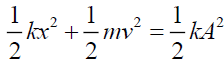.While, the total work (W) done by these variable spring forces on the cosmological objects when it moves from (-A→+A) is zero, where A is the amplitude of the simple harmonic motion with no energy transfer or loss leaving that isolated system . The instantaneous power (P) of the oscillator along these paths is defined by the rate of energy transfer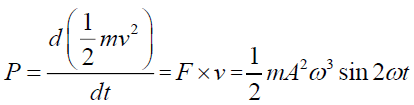where kinetic energy is the energy transfer method in the simple harmonic motion . Thus, no gravitational waves produced when the spring force vanishes at the equilibrium position of the spring when the cosmological body (oscillator) is on the axis of the wave. Along the wavy path the velocity of the oscillator (cosmological object) varies inversely with the acting variable spring force. The oscillator passes the axis of the wave with maximum velocity when the spring force vanishes and comes to instantaneous rest at the crests and the troughs at the maximum value of the spring force. Thus, for each gravitational wave produced by the cosmological body along the wavy path by certain velocity during compression there is identical one (same amplitude and frequency) produced by the same velocity in opposite direction during stretching. Accordingly, the gravitational waves produced by the oscillator during compression destruct those produced during stretching preserving self-sustaining of frictionless system. Gravitational waves produced by the oscillator just before or after passing the wave axis leave the oscillator system (energy loss) as they pushed by the other cosmological object that acts on the oscillator by the spring force in its direction along its elliptical path. Afterwards, these gravitational waves re-enter the oscillator system (energy gain) by the oscillator once again preserving energy of the renewable oscillator system. FIG.10 shows the generated gravitational waves by the moon (silver arrows) and the earth (blue arrows) along their paths, and the energy loss from the wavy path of the moon by the earth (red arrows) that re-enter the wavy path again (energy gain) by the moon (green arrows) as detected from the sun (yellow).Accordingly, theory of the relative image predicts that gravitational waves observations of the cosmological bodies expresses those produced by the oscillator just before and after passing the wave axis (just before and after the spring force vanishes) only. Thus, observational gravitational waves generated by an oscillator whose simple harmonic motion expressed by ( x= Asinωt ) is predicted to be observed only when time (t),where T is the periodic time of the simple harmonic motion. Such perception for the detected gravitational waves provided by theory of the relative image is consistent with their tiny detected observations. For instance, the velocity of the moon along its wavy path just before or after passing the elliptical path of the earth around the sun as detected from the sun in the perpendicular direction to the elliptical path is 1023 m/s. At these positions, the generated gravitational waves by the moon is pushed away (energy loss) the wavy path of the moon by the earth and re-enter (energy gain) the wavy path of the moon once again by the moon itself on its consequent crossing the elliptical path. Thus, if the power of the detected gravitational waves is 200 watt, then the force drives the moon at these positions is (F=Power/velocity).Accordingly, the positions at which the gravitational waves were detected are distant by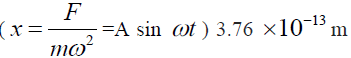from the elliptical path of the earth around the sun, when time, where T is the periodic time of the simple harmonic motion as postulated by theory of the relative image.

Static model of our infinite universe

Theory of the relative image gives new insights into the working of the Universe. It refutes what has been postulated by either of the Newtonian and general relativity that the gravitational forces acting on the cosmological bodies are constant and centripetal always attractive [25,26]. Conversely, it proves that the gravitational forces that act on the cosmological bodies are variable elastic restoring spring forces under which the cosmological bodies move along wavy paths preserving the fixed distances between them which are the amplitudes of their simple harmonic motions. However, equilibrium of the cosmological body in any of its relative images has been clarified inseparable from the equilibrium of the whole universe as one unit. All the contents of this unit are bounded by an integrated system of variable elastic restoring (spring) forces of simple harmonic motions (seem constant only due to the observer position). These contents (the cosmological objects) are driven by these variable spring forces along wavy paths, with velocity decrease gradually until they come to the instantaneous rest at the maximum amplitude of the motion then the spring forces reversed in opposite direction with maximum value and change the direction of motion with maximum velocity once again, like oxygen when revives the heart after exhaling to keep life. Statics generates motion that decreases gradually and faints again until revives and so on. Accordingly, velocities of all the contents of our universe are not constant but accelerated and then decelerated in a frictionless isolated system of permanent self-sustaining. Such acceleration and deceleration expresses the balance between the even distribution of the matter along the spacetime. Despite of the relative path length that observed differs from image to another for the same motion; the spaces between objects (Amplitudes of the wavy paths) are fixed in all these relative images reflecting a precise system. Thus, theory of the relative image proves that matter and spacetime mutually interact preserving their even distribution and consequently the static of the universe as a one unit. This fact refutes the expanding model as a proposed model for the universe. Conversely, theory of the relative image proves that our universe is like a self-sustaining being always static, while all its inner organs never stop through efficient cycle of acceleration and deceleration without energy loss (path-decay) so that no absolute rest along the spacetime. Such cycles of acceleration and deceleration while the cosmological objects are under compression and then stretching affect the wavelengths of the transmitted gravitational waves across the universe in the direction of motion of these bodies which make it hard to be detected. Furthermore, every gravitational wave has its reversible for self-destruction as shown before (Figure. 7) so that no influences occur on the fixed space between objects (Amplitudes of the wavy paths) sustaining the static of the universe. In addition, our suggested selfsustaining static universe of none absolute rest along its spacetime explains as previously shown the observations of the intergalactic redshift, emitted radiations by the black holes and the cosmic microwave background radiation that misled the latter to propose the expansion model for our universe after the Big-Bang.

### Discussion

Figure 10: The generated gravitational waves by the Moon (silver arrows) along its wavy path around the Earth and energy loss by the Earth (blue arrows) and energy gain by the Moon (green arrows) as detected from the Sun (yellow).

### Conclusion

It proves that all paths of the celestial bodies along the spacetime are fixed never change and galaxies are not receding away from each other. Our universe has been designed through precise balance of the matter distribution along the spacetime. Such balance achieves the unique frictionless, renewable, isolated system of permanent self-sustaining so that nothing resembles our universe.

### References

Select your language of interest to view the total content in your interested language

###### Citations : 214

Journal of Space Exploration received 214 citations as per Google Scholar report

#### Indexed In

• Index Copernicus
• Open J Gate
• China National Knowledge Infrastructure (CNKI)
• Cosmos IF
• Directory of Research Journal Indexing (DRJI)
• Secret Search Engine Labs

View More

#### For Librarians